Using Communications Toolbox™ you can implement fading channels using objects or blocks. Rayleigh and Rician fading channels are useful models of real-world phenomena in wireless communications. These phenomena include multipath scattering effects, time dispersion, and Doppler shifts that arise from relative motion between the transmitter and receiver. This section gives a brief overview of fading channels and describes how to implement them using the toolbox.

This figure depicts direct and major reflected paths between a stationary radio transmitter and a moving receiver. The shaded shapes represent reflectors such as buildings.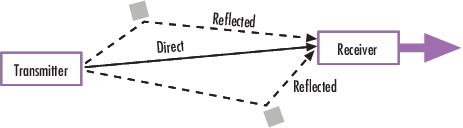The major paths result in the arrival of delayed versions of the signal at the receiver. In addition, the radio signal undergoes scattering on a local scale for each major path. Such local scattering typically results from reflections off objects near the mobile. These irresolvable components combine at the receiver and cause a phenomenon known as multipath fading. Due to this phenomenon, each major path behaves as a discrete fading path. Typically, the fading process is characterized by a Rayleigh distribution for a non line-of-sight path and a Rician distribution for a line-of-sight path.

The relative motion between the transmitter and receiver causes Doppler shifts. Local scattering typically comes from many angles around the mobile. This scenario causes a range of Doppler shifts, known as the Doppler spectrum. The maximum Doppler shift corresponds to the local scattering components whose direction exactly opposes the trajectory of the mobile.

The channel filter applies path gains to the input signal, `Signal in`. The path gains are configured based on settings chosen in the fading channel object or block.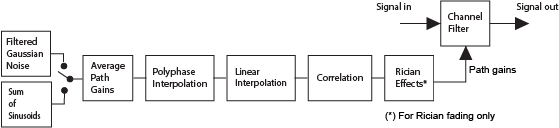These blocks and objects enable you to model SISO or MIMO fading channels.

#### Implement Fading Channel Using an Object

A baseband channel model for multipath propagation scenarios that you implement using objects includes:

• N discrete fading paths. Each path has its own delay and average power gain. A channel for which N = 1 is called a frequency-flat fading channel. A channel for which N > 1 is experienced as a frequency-selective fading channel by a signal of sufficiently wide bandwidth.

• A Rayleigh or Rician model for each path.

• Default channel path modeling using a Jakes Doppler spectrum, with a maximum Doppler shift that can be specified. Other types of Doppler spectra allowed (identical or different for all paths) include: flat, restricted Jakes, asymmetrical Jakes, Gaussian, bi-Gaussian, rounded, and bell.

If the maximum Doppler shift is set to 0 or omitted during the construction of a channel object, then the object models the channel as static. For this configuration, the fading does not evolve with time and the Doppler spectrum specified has no effect on the fading process.

Some additional information about typical values for delays and gains is in Choosing Realistic Channel Property Values.

#### Implement Fading Channel Using a Block

The Channels block library includes MIMO and SISO fading blocks that can simulate real-world phenomena in mobile communications. These phenomena include multipath scattering effects, in addition to Doppler shifts that arise from relative motion between the transmitter and receiver.

Tip

To model a channel that involves both fading and additive white Gaussian noise, use a fading channel block followed by an AWGN Channel block.

The MIMO Fading Channel and SISO Fading Channel blocks can be set to model Rayleigh or Rician fading distributions of the channel. Based on the type of signal path, choose the fading distribution to use.

Direct line-of-sight path from transmitter to receiver `Rician`
One or more major reflected paths from transmitter to receiver `Rayleigh`

You can use a single instance of a fading channel block configured for Rayleigh fading distribution to model multiple major reflected paths simultaneously.

Choosing appropriate block parameters for your situation is important. For more information, see Choosing Realistic Channel Property Values, and the MIMO Fading Channel and SISO Fading Channel block reference pages.

You can view the characteristics of a fading channel using channel visualization tools. For more information, see Channel Visualization.

A communications system involving a fading channel usually requires components that compensate for the fading response. Typical approaches to compensate for fading include:

• Differential modulation or a one-tap equalizer helps compensate for a frequency-flat fading channel. For information about implementing differential modulation, see the M-DPSK Modulator Baseband block reference page.

• An equalizer with multiple taps helps compensate for a frequency-selective fading channel. See Equalization for more information.

### Methodology for Simulating Multipath Fading Channels

The Rayleigh and Rician multipath fading channel simulators in Communications Toolbox use the band-limited discrete multipath channel model of section 9.1.3.5.2 in . This implementation assumes that the delay power profile and the Doppler spectrum of the channel are separable . The multipath fading channel is therefore modeled as a linear finite impulse-response (FIR) filter. Let $\left\{{s}_{i}\right\}$ denote the set of samples at the input to the channel. Then the samples $\left\{{y}_{i}\right\}$ at the output of the channel are related to $\left\{{s}_{i}\right\}$ through:

`${y}_{i}=\sum _{n=-{N}_{1}}^{{N}_{2}}{s}_{i-n}{g}_{n}$`

where $\left\{{g}_{n}\right\}$ is the set of tap weights given by:

In the equations:

• ${T}_{s}$ is the input sample period to the channel.

• $\left\{{\tau }_{k}\right\}$, where $1\le k\le K$, is the set of path delays. K is the total number of paths in the multipath fading channel.

• $\left\{{a}_{k}\right\}$, where $1\le k\le K$, is the set of complex path gains of the multipath fading channel. These path gains are uncorrelated with each other.

• ${N}_{1}$ and ${N}_{2}$ are chosen so that $|{g}_{n}|$ is small when n is less than $-{N}_{1}$ or greater than ${N}_{2}$.

Two techniques, filtered Gaussian noise and sum-of-sinusoids, are used to generate the set of complex path gains, ${a}_{k}$.

Each path gain process ${a}_{k}$ is generated by the following steps:

Filtered Gaussian Noise Technique

1. A complex uncorrelated (white) Gaussian process with zero mean and unit variance is generated in discrete time.

2. The complex Gaussian process is filtered by a Doppler filter with frequency response $H\left(f\right)=\sqrt{S\left(f\right)}$, where $S\left(f\right)$ denotes the desired Doppler power spectrum.

3. The filtered complex Gaussian process is interpolated so that its sample period is consistent with the sample period of the input signal. A combination of linear and polyphase interpolation is used.

Sum-of-sinusoids Technique

1. Mutually uncorrelated Rayleigh fading waveforms are generated using the method described in , where i = 1 corresponds to the in-phase component and i = 2 corresponds to the quadrature component.

`$\begin{array}{c}{z}_{k}\left(t\right)={\mu }_{k}^{\left(1\right)}\left(t\right)+j{\mu }_{k}^{\left(2\right)}\left(t\right),\text{ }k=1,2,\dots ,K\\ {\mu }_{k}^{\left(i\right)}\left(t\right)=\sqrt{\frac{2}{{N}_{k}}}\sum _{n=1}^{{N}_{k}}\mathrm{cos}\left(2\pi {f}_{k,n}^{\left(i\right)}t+{\theta }_{k,n}^{\left(i\right)}\right),\text{ }i=1,2\end{array}$`

Where

• Nk specifies the number of sinusoids used to model a single path.

• ${f}_{k,n}^{\left(i\right)}$ is the discrete Doppler frequency and is calculated for each sinusoid component within a single path.

• ${\theta }_{k,n}^{\left(i\right)}$ is the phase of the nth component of ${\mu }_{k}^{\left(i\right)}$ and is an i.i.d. random variable having a uniform distribution over the interval $\left(0,2\pi \right]$.

• t is the fading process time.

When modeling a Jakes Doppler spectrum, the discrete Doppler frequencies, ${f}_{k,n}^{\left(i\right)}$, with maximum shift fmax are given by

`$\begin{array}{c}{f}_{k,n}^{\left(i\right)}={f}_{\mathrm{max}}\mathrm{cos}\left({\alpha }_{k,n}^{\left(i\right)}\right)\\ ={f}_{\mathrm{max}}\mathrm{cos}\left[\frac{\pi }{2{N}_{k}}\left(n-\frac{1}{2}\right)+{\alpha }_{k,0}^{\left(i\right)}\right]\end{array}$`

where

`${\alpha }_{k,0}^{\left(i\right)}\triangleq {\left(-1\right)}^{i-1}\frac{\pi }{4{N}_{k}}\cdot \frac{k}{K+2},\text{ }i=1,\text{\hspace{0.17em}}2\text{\hspace{0.17em}}\text{\hspace{0.17em}}\text{and}\text{\hspace{0.17em}}\text{\hspace{0.17em}}k=1,2,\dots ,K$`
2. To advance the fading process in time, an initial time parameter, tinit, is introduced. The fading waveforms become

`${\mu }_{k}^{\left(i\right)}\left(t\right)=\sqrt{\frac{2}{{N}_{k}}}\sum _{n=1}^{{N}_{k}}\mathrm{cos}\left(2\pi {f}_{k,n}^{\left(i\right)}\left(t+{t}_{init}\right)+{\theta }_{k,n}^{\left(i\right)}\right),\text{ }i=1,2$`

When tinit = 0, the fading process starts at time zero. A positive value of tinit advances the fading process relative to time zero while maintaining its continuity.

3. Channel fading samples are generated using the GMEDS1  algorithm.

Calculate Complex Coefficients

The complex process resulting from either technique, ${z}_{k}$, is scaled to obtain the correct average path gain. In the case of a Rayleigh channel, the fading process is obtained as:

`${a}_{k}=\sqrt{{\Omega }_{k}}{z}_{k}$`

where

`${\Omega }_{k}=E\left[{|{a}_{k}|}^{2}\right]$`

In the case of a Rician channel, the fading process is obtained as:

`${a}_{k}=\sqrt{{\Omega }_{k}}\left[\frac{{z}_{k}}{\sqrt{{K}_{r,k}+1}}+\sqrt{\frac{{K}_{r,k}}{{K}_{r,k}+1}}{e}^{j\left(2\pi {f}_{d,LOS,k}t+{\theta }_{LOS,k}\right)}\right]$`

where ${K}_{r,k}$ is the Rician K-factor of the kth path, ${f}_{d,LOS,k}$ is the Doppler shift of the line-of-sight component of the kth path (in Hz), and ${\theta }_{LOS,k}$ is the initial phase of the line-of-sight component of the kth path (in rad).

At the input to the band-limited multipath channel model, the transmitted symbols must be oversampled by a factor at least equal to the bandwidth expansion factor introduced by pulse shaping. For example, if sinc pulse shaping is used, for which the bandwidth of the pulse-shaped signal is equal to the symbol rate, then the bandwidth expansion factor is 1, and at least one sample per symbol is required at the input to the channel. If a raised cosine (RC) filter with a factor more than 1 is used, for which the bandwidth of the pulse-shaped signal is equal to twice the symbol rate, then the bandwidth expansion factor is 2, and at least two samples per symbol are required at the input to the channel.

#### Channel Filter Model Characteristics

The channel filter implements a fractional delay (FD) finite impulse response (FIR) bandpass filter with a length of 16 coefficients for each candidate fractional delay at 0, 0.02, 0.04, …, 0.98.

Each discrete path is rounded to its nearest candidate fractional delay, so the delay error limit is 1% of the sample time. To achieve a group delay bandwidth exceeding 80% and a magnitude bandwidth exceeding 90%, the algorithm selects the optimal FIR coefficient values for each fractional delay, while satisfying the following criteria:

• Group delay ripple ≤ 10%

• Magnitude ripple ≤ 2 dB

• Magnitude bandedge attenuation = 3 dB

The plots show bandwidths that satisfy the design criteria for group delay ripple, magnitude ripple, and magnitude bandedge attenuation.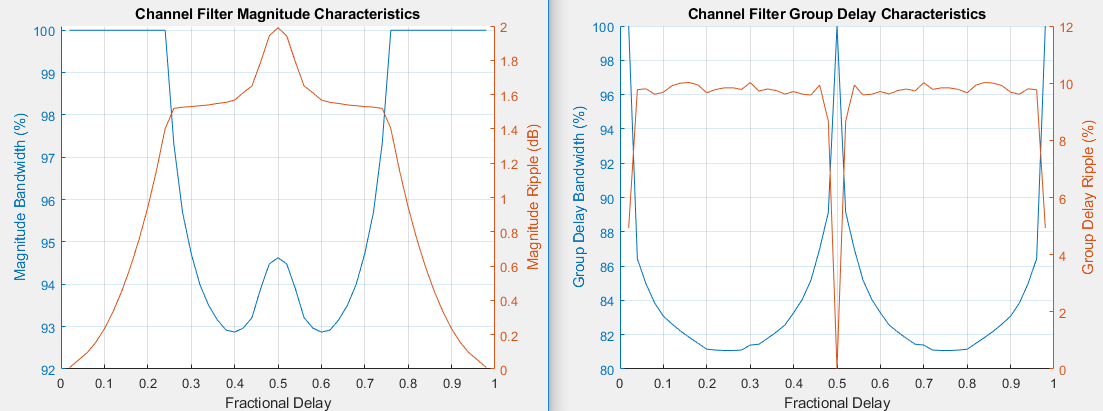#### References

 Jeruchim, M. C., Balaban, P., and Shanmugan, K. S., Simulation of Communication Systems, Second Edition, New York, Kluwer Academic/Plenum, 2000.

 Pätzold, Matthias, Cheng-Xiang Wang, and Bjorn Olav Hogstand. “Two New Sum-of-Sinusoids-Based Methods for the Efficient Generation of Multiple Uncorrelated Rayleigh Fading Waveforms.” IEEE Transactions on Wireless Communications. Vol. 8, Number 6, 2009, pp. 3122–3131.

Communications Toolbox models a fading channel as a linear FIR filter. Filtering a signal using a fading channel involves these steps:

1. Create a channel object that describes the channel that you want to use. A channel object is a type of MATLAB variable that contains information about the channel, such as the maximum Doppler shift.

2. Adjust properties of the channel object, if necessary, to tailor it to your needs. For example, you can change the path delays or average path gains.

3. Apply the channel object to your signal using calling the object.

This section describes how to define, inspect, and manipulate channel objects. The topics are:

#### Creating Channel Objects

To create a fading channel object suitable for your modeling situation, select one of these System objects.

FunctionObjectSituation Modeled

`comm.RayleighChannel`

Rayleigh fading channel objectOne or more major reflected paths

`comm.RicianChannel`

Rician fading channel objectOne direct line-of-sight path, possibly combined with one or more major reflected paths

For example, this command creates a channel object representing a Rayleigh fading channel that acts on a signal sampled at 100,000 Hz. The maximum Doppler shift of the channel is 130 Hz.

```rayChan1 = comm.RayleighChannel('SampleRate',1e5,... 'MaximumDopplerShift',130); % Rayleigh fading channel object ```

To learn how to call the `rayChan1` fading channel object to filter the transmitted signal through the channel, see Using Fading Channels.

#### Duplicate and Copy Objects

You can also create another object by duplicating an existing object and then adjust the properties of the new object, if necessary. To duplicate an object always use the `clone` function such as:

`rayChan2 = clone(rayChan1); % Copy rayChan1 to create an independent rayChan2.`

instead of `rayChan2 = rayChan1`. The `clone` command creates a copy of `rayChan1` that is independent of `rayChan1`. By contrast, the command ```rayChan2 = rayChan1``` creates `rayChan2` as merely a reference to `rayChan1`, so that `rayChan1` and `rayChan2` always have indistinguishable content.

#### Displaying and Changing Object Properties

A channel object has numerous properties that record information about the channel model, about the state of a channel that has already filtered a signal, and about the channel operation on a future signal.

You can view the properties in these ways:

• To view all properties of a channel object, enter the object name in the Command Window.

• You can view a property of a channel object or assign the value to a variable by entering the object name followed by a dot (period), followed by the name of the property.

You can change the writable properties of a channel object in these ways:

• To change the default value of a channel object property, enter the desired value in the object creation syntax.

• To change the value of a writeable property of a channel object, issue an assignment statement that uses dot notation on the channel object. More specifically, dot notation means an expression that consists of the object name, followed by a dot, followed by the name of the property.

Display Rayleigh Channel Object Properties

Create a Rayleigh channel object. Display the object to show the properties initialized by default and the ones specified when creating the object. Entering `rayChan` displays all properties of the channel object. Some of the properties values were assigned when the object was created, while other properties have default values. For more information about specific channel properties, see the reference page for the `comm.RayleighChannel` object.

```rayChan = comm.RayleighChannel('SampleRate',1e5,'MaximumDopplerShift',130); rayChan % View all the properties```
```rayChan = comm.RayleighChannel with properties: SampleRate: 100000 PathDelays: 0 AveragePathGains: 0 NormalizePathGains: true MaximumDopplerShift: 130 DopplerSpectrum: [1x1 struct] Show all properties ```
`g = rayChan.AveragePathGains % Retrieve the AveragePathGains property of rayChan`
```g = 0 ```

A Rician fading channel object has an additional property that does not appear for a Rayleigh fading channel object, namely, a scalar `KFactor` property. For more information about Rician channel properties, see the reference page for the `comm.RicianChannel` object.

Change Rician Channel Object Properties

Create a Rician channel object. The default setting for the `Visualization` property is `'Off'`. Changing the default setting to `'Impulse response'` generates an impulse response plot of the output signal when the object is called. The output displays a subset of all the properties of the channel object. Select `all properties` to see the complete set of properties for `ricChan`.

```ricChan= comm.RicianChannel; % Create object ricChan.Visualization = 'Impulse response' % Enables the impulse response channel visualization```
```ricChan = comm.RicianChannel with properties: SampleRate: 1 PathDelays: 0 AveragePathGains: 0 NormalizePathGains: true KFactor: 3 DirectPathDopplerShift: 0 DirectPathInitialPhase: 0 MaximumDopplerShift: 1.0000e-03 DopplerSpectrum: [1x1 struct] Show all properties ```

#### Relationships Among Channel Object Properties

Some properties of a channel object are related to each other such that when one property's value changes, another property's value must change in some corresponding way to keep the channel object consistent. For example, if you change the vector length of `PathDelays`, then the value of `AveragePathGains` must change so that its vector length equals that of the new value of `PathDelays`. This is because the length of each of the two vectors equals the number of discrete paths of the channel. For details about linked properties and an example, see `comm.RayleighChannel` or `comm.RicianChannel`.

### Specify Doppler Spectrum of Fading Channel

The Doppler spectrum of a channel object is specified through its `DopplerSpectrum` property. The value of this property must be either:

• A Doppler spectrum structure. In this case, the same Doppler spectrum applies to each path of the channel object.

• A cell array of Doppler spectrum structures of the same length as the `PathDelays` vector property. In this case, the Doppler spectrum of each path is given by the corresponding Doppler spectrum structure in the vector.

• When the vector length of the `PathDelays` property is increased, the length of `DopplerSpectrum` is automatically increased to match the length of `PathDelays`, by appending Jakes Doppler spectrum structures.

• If the length of the `PathDelays` vector property is decreased, the length of `DopplerSpectrum` is automatically decreased to match the length of `PathDelays`, by removing the last Doppler spectrum structures.

A Doppler spectrum structure contains the properties used to characterize the Doppler spectrum, but the maximum Doppler shift is a property of the channel object. This section describes how to create and manipulate Doppler spectrum structures, and how to assign them to the `DopplerSpectrum` property of channel objects.

#### Create a Doppler Spectrum Structure

To create Doppler spectrum structures, use the `doppler` function. The sole purpose of the `doppler` function is to create Doppler spectrum structures used to specify the value of the `DopplerSpectrum` property of channel objects. Select from the following:

• `doppler('Jakes')`

• `doppler('Flat')`

• `doppler('Rounded', ...)`

• `doppler('Bell', ...)`

• `doppler('Asymmetric Jakes', ...)`

• `doppler('Restricted Jakes', ...)`

• `doppler('Gaussian', ...)`

• `doppler('BiGaussian', ...)`

For example, a Gaussian spectrum with a normalized (by the maximum Doppler shift of the channel) standard deviation of 0.1, can be created as:

`dopp1 = doppler('Gaussian',0.1);`

Note

When creating a Doppler spectrum structure, consider the following dependencies:

• If you assign a single Doppler spectrum structure to `DopplerSpectrum`, all paths have the same specified Doppler spectrum.

• If the `FadingTechnique` property is ```'Sum of sinusoids'```, `DopplerSpectrum` must be `doppler('Jakes')`;

• If you assign a row cell array of different Doppler spectrum structures to `DopplerSpectrum`, each path has the Doppler spectrum specified by the corresponding structure in the cell array. In this case, the length of `DopplerSpectrum` must be equal to the length of `PathDelays`.

• To generate C code, specify `DopplerSpectrum` to a single Doppler spectrum structure.

#### View and Change Doppler Spectrum Structure Properties

Create a Doppler spectrum structure by specifying the type of Doppler spectrum as `'Rounded'`, then modify settings of the polynomial type

A rounded Doppler spectrum structure with default properties is created and displayed, and the third element of the `Polynomial` field is modified.

`doppRound = doppler('Rounded')`
```doppRound = struct with fields: SpectrumType: 'Rounded' Polynomial: [1 -1.7200 0.7850] ```

Adjust the third coefficient of the polynomial.

`doppRound.Polynomial(3) = 0.825`
```doppRound = struct with fields: SpectrumType: 'Rounded' Polynomial: [1 -1.7200 0.8250] ```

Be aware that it is possible to modify a Doppler spectrum structure to an invalid configuration. Validation of the Doppler spectrum structure settings is performed when the structure is used by a fading channel object. The `doppRound` spectrum structure defined above is valid.

`ricianCh = comm.RicianChannel('DopplerSpectrum',doppRound)`
```ricianCh = comm.RicianChannel with properties: SampleRate: 1 PathDelays: 0 AveragePathGains: 0 NormalizePathGains: true KFactor: 3 DirectPathDopplerShift: 0 DirectPathInitialPhase: 0 MaximumDopplerShift: 1.0000e-03 DopplerSpectrum: [1x1 struct] Show all properties ```

#### Use Doppler Spectrum Structures Within Channel Objects

The `DopplerSpectrum` property of a channel object can be changed by assigning to it a Doppler spectrum structure or a vector of Doppler spectrum structures.

#### Create Rayleigh Channel with Flat Doppler Spectrum

This example illustrates how to change the default Jakes Doppler spectrum of a constructed Rayleigh channel object to a flat Doppler spectrum.

Create a Rayleigh channel object

Set the sample rate to 9600 Hz and the maximum Doppler shift to 100 Hz.

`rayChan = comm.RayleighChannel('SampleRate',9600, 'MaximumDopplerShift',100)`
```rayChan = comm.RayleighChannel with properties: SampleRate: 9600 PathDelays: 0 AveragePathGains: 0 NormalizePathGains: true MaximumDopplerShift: 100 DopplerSpectrum: [1x1 struct] Show all properties ```
`rayChan.DopplerSpectrum`
```ans = struct with fields: SpectrumType: 'Jakes' ```

Modify the Doppler spectrum

Create a flat Doppler spectrum structure and assign it in the `rayChan` object.

`doppFlat = doppler('Flat')`
```doppFlat = struct with fields: SpectrumType: 'Flat' ```
`rayChan.DopplerSpectrum = doppFlat`
```rayChan = comm.RayleighChannel with properties: SampleRate: 9600 PathDelays: 0 AveragePathGains: 0 NormalizePathGains: true MaximumDopplerShift: 100 DopplerSpectrum: [1x1 struct] Show all properties ```
`rayChan.DopplerSpectrum`
```ans = struct with fields: SpectrumType: 'Flat' ```

#### Create Rician Channel with Gaussian Doppler Spectrum

This example shows how to change the default Jakes Doppler spectrum of a constructed Rician channel object to a Gaussian Doppler spectrum with normalized standard deviation of 0.3, and subsequently display the `DopplerSpectrum` property, and change the value of the normalized standard deviation to 1.1.

Create a Rician channel object

Set the sample rate to 9600 Hz, the maximum Doppler shift to 100 Hz, and K factor to 2.

`ricChan = comm.RicianChannel('SampleRate',9600, 'MaximumDopplerShift',100, 'KFactor',2)`
```ricChan = comm.RicianChannel with properties: SampleRate: 9600 PathDelays: 0 AveragePathGains: 0 NormalizePathGains: true KFactor: 2 DirectPathDopplerShift: 0 DirectPathInitialPhase: 0 MaximumDopplerShift: 100 DopplerSpectrum: [1x1 struct] Show all properties ```
`ricChan.DopplerSpectrum`
```ans = struct with fields: SpectrumType: 'Jakes' ```

Modify the Doppler spectrum

Create a Gaussian Doppler spectrum structure with normalized standard deviation of 0.3 and assign it in the `ricChan` object.

`doppGaus = doppler('Gaussian',0.3)`
```doppGaus = struct with fields: SpectrumType: 'Gaussian' NormalizedStandardDeviation: 0.3000 ```
`ricChan.DopplerSpectrum = doppGaus`
```ricChan = comm.RicianChannel with properties: SampleRate: 9600 PathDelays: 0 AveragePathGains: 0 NormalizePathGains: true KFactor: 2 DirectPathDopplerShift: 0 DirectPathInitialPhase: 0 MaximumDopplerShift: 100 DopplerSpectrum: [1x1 struct] Show all properties ```
`ricChan.DopplerSpectrum`
```ans = struct with fields: SpectrumType: 'Gaussian' NormalizedStandardDeviation: 0.3000 ```
```ricChan.DopplerSpectrum.NormalizedStandardDeviation = 1.1; ricChan.DopplerSpectrum```
```ans = struct with fields: SpectrumType: 'Gaussian' NormalizedStandardDeviation: 1.1000 ```

#### Create Rayleigh Channel Using Independent Doppler Spectrum

This example illustrates how to change the default Jakes Doppler spectrum of a constructed three-path Rayleigh channel object to a cell array of different Doppler spectra, and then change the properties of the Doppler spectrum of the third path.

Create a Rayleigh channel object

Set the sample rate to 9600 Hz, the maximum Doppler shift to 100 Hz, and specify path delays of `0`, `1e-4`, and `2.1e-4 seconds`.

`rayChan = comm.RayleighChannel('SampleRate',9600, 'MaximumDopplerShift',100, 'PathDelays',[0 1e-4 2.1e-4])`
```rayChan = comm.RayleighChannel with properties: SampleRate: 9600 PathDelays: [0 1.0000e-04 2.1000e-04] AveragePathGains: 0 NormalizePathGains: true MaximumDopplerShift: 100 DopplerSpectrum: [1x1 struct] Show all properties ```
`rayChan.DopplerSpectrum`
```ans = struct with fields: SpectrumType: 'Jakes' ```

Modify the Doppler spectrum

Specify the `DopplerSpectrum` property as a cell array with an independent Doppler spectrum for each path.

`rayChan.DopplerSpectrum = {doppler('Flat') doppler('Flat') doppler('Rounded')}`
```rayChan = comm.RayleighChannel with properties: SampleRate: 9600 PathDelays: [0 1.0000e-04 2.1000e-04] AveragePathGains: 0 NormalizePathGains: true MaximumDopplerShift: 100 DopplerSpectrum: {[1x1 struct] [1x1 struct] [1x1 struct]} Show all properties ```
`rayChan.DopplerSpectrum{:}`
```ans = struct with fields: SpectrumType: 'Flat' ```
```ans = struct with fields: SpectrumType: 'Flat' ```
```ans = struct with fields: SpectrumType: 'Rounded' Polynomial: [1 -1.7200 0.7850] ```

Change the `Polynomial` property for the third path.

`rayChan.DopplerSpectrum{3}.Polynomial = [1 -1.21 0.7]`
```rayChan = comm.RayleighChannel with properties: SampleRate: 9600 PathDelays: [0 1.0000e-04 2.1000e-04] AveragePathGains: 0 NormalizePathGains: true MaximumDopplerShift: 100 DopplerSpectrum: {[1x1 struct] [1x1 struct] [1x1 struct]} Show all properties ```
`rayChan.DopplerSpectrum{:}`
```ans = struct with fields: SpectrumType: 'Flat' ```
```ans = struct with fields: SpectrumType: 'Flat' ```
```ans = struct with fields: SpectrumType: 'Rounded' Polynomial: [1 -1.2100 0.7000] ```

### Configure Channel Objects

Before you filter a signal using a channel object, make sure that the properties of the channel have suitable values for the situation you want to model. This section offers some guidelines to help you choose realistic values that are appropriate for your modeling needs. The topics are

The syntaxes for viewing and changing values of properties of channel objects are described in Specifying a Fading Channel.

#### Choose Realistic Channel Property Values

Here are some tips for choosing property values that describe realistic channels:

Path Delays

• By convention, the first delay is typically set to zero. The first delay corresponds to the first arriving path.

• For indoor environments, path delays after the first are typically between 1e-9 seconds and 1e-7 seconds.

• For outdoor environments, path delays after the first are typically between 1e-7 seconds and 1e-5 seconds. Large delays in this range might correspond, for example, to an area surrounded by mountains.

• The ability of a signal to resolve discrete paths is related to its bandwidth. If the difference between the largest and smallest path delays is less than about 1% of the symbol period, then the signal experiences the channel as if it had only one discrete path.

Average Path Gains

• The average path gains in the channel object indicate the average power gain of each fading path. In practice, an average path gain value is a large negative dB value. However, computer models typically use average path gains in the range of [-20, 0] dB.

• The dB values in a vector of average path gains often decay roughly linearly as a function of delay, but the specific delay profile depends on the propagation environment.

• To ensure that the expected total power of the combined path gains is 1, you can normalize path gains via the `NormalizePathGains` property of the channel object.

Maximum Doppler Shifts

• Doppler shifts are specified in terms of the relative speed between a transmitter and a receiver. The maximum Doppler shift in Hertz, fd = vfc. In the formula, v is the relative speed in m/s, f is the transmission carrier frequency in Hertz, and c is the speed of light (3×108 m/s). The relative speed is a magnitude with no directional information.

• Apply the maximum Doppler shift formula assuming a transmission carrier frequency of 900 MHz, a car moving at freeway speed, and a walking pedestrian. A signal transmitted from a car moving at freeway speed to a stationary receiver would experience a maximum Doppler shift of approximately 80 Hz. A signal transmitted from a mobile held by a walking pedestrian to a stationary receiver would experience a maximum Doppler shift of approximately 4 Hz.

• A maximum Doppler shift of 0 corresponds to a static channel that comes from a Rayleigh or Rician distribution.

Doppler Spectrum

• The Doppler spectrum used for the channel paths must be outputs of the form returned from the `doppler` function.

• Options for the spectrum type are specified by the `specType` input to the `doppler` function.

• The Rician K-factor specifies the ratio of specular-to-diffuse power for a direct line-of-sight path. The ratio is expressed linearly, not in dB.

• For Rician fading, the K-factor is typically in the range [1, 10].

• A K-factor of 0 corresponds to Rayleigh fading.

Line-of-Sight (LOS) Path Doppler Shift for Rician Fading Channels

• The Rician LOS path Doppler shift, also known as direct path Doppler shift, specifies the relative motion of the LOS path between a transmitter and a receiver.

• The Rician LOS path Doppler shift in Hertz, fd_los = (uw) × fc. In the formula, (uw) is the dot product of vectors u and w, u is the normalized LOS path from the transmitter to the receiver, w is the velocity of the receiver relative to transmitter, f is the transmission carrier frequency in Hertz, and c is the speed of light (3×108 m/s).

• Apply this formula for a transmission carrier frequency of 900 MHz at a specified relative velocity. For a signal from a transmitter at the coordinate origin that reaches a receiver at the coordinate [100 100 0], where the relative velocity between the transmitter and receiver w = [3 -6 0.1]. The LOS path Doppler shift is 4.25 Hz.

Doppler Spectrum Parameters

• See the `doppler` reference page for the respective Doppler spectrum structures for descriptions of the parameters and their significance.

#### Configure Channel Objects Based on Simulation Needs

Tips for configuring a channel object to customize the filtering process:

• If your data is partitioned into a series of vectors (that you process within a loop, for example), you can call the channel object multiple times (in each iteration within a loop). The state information of the channel is updated and saved after each invocation. The channel output is irrelevant to how the data is partitioned (vector length).

• If want the channel output to be repeatable, choose the seed option for the `RandomStream` property of the channel object. To repeat the output, call the `reset` object function to reset both the internal filters and the internal random number generator.

• If you want to model discontinuously transmitted data, set the `FadingTechnique` property to `'Sum of sinusoids'` and the `InitialTimeSource` property to ```'Input port'``` for the channel object. When calling the object, specify the start time of each data vector/frame to be processed by the channel via an input.

• If you want to normalize the fading process so that the expected value of the path gains' total power is 1 (the channel does not contribute additional power gain or loss), set the `NormalizePathGains` property of the channel object to `true`.

After you have created a channel object as described in Specifying a Fading Channel, you can call the object to pass a signal through the channel. Provide the signal as an input argument to the channel object. At the end of the filtering operation, the channel object retains its state so that you can find out the final path gains or the total number of samples that the channel has processed by calling the info object function with the object as the input argument.

For an example that illustrates the basic syntax and state retention, see Power of a Faded Signal.

To visualize the characteristics of the channel, set the `Visualization` property to `'Impulse response'`, `'Frequency response'`, or `'Doppler spectrum'`. For more information, see Channel Visualization.

#### Visualize Three-Path Rayleigh Channel

This example shows you how to visualize impulse response of a channel.

Create a channel object

While creating the channel object, use name-value pairs to set the `Visualization` property

to `'Impulse response'`.

```rayChan = comm.RayleighChannel('SampleRate',100000,'MaximumDopplerShift',130,... 'PathDelays',[0 1.5e-5 3.2e-5],'AveragePathGains',[0, -3, -3],... 'Visualization','Impulse response'); ```

Generate a bit stream and create a modulator object. Modulate the bit stream and pass the

modulated DBPSK signal through the channel by calling the channel object.

```tx = randi([0 1],500,1); dbspkMod = comm.DBPSKModulator; dpskSig = dbspkMod(tx); y = rayChan(dpskSig); ```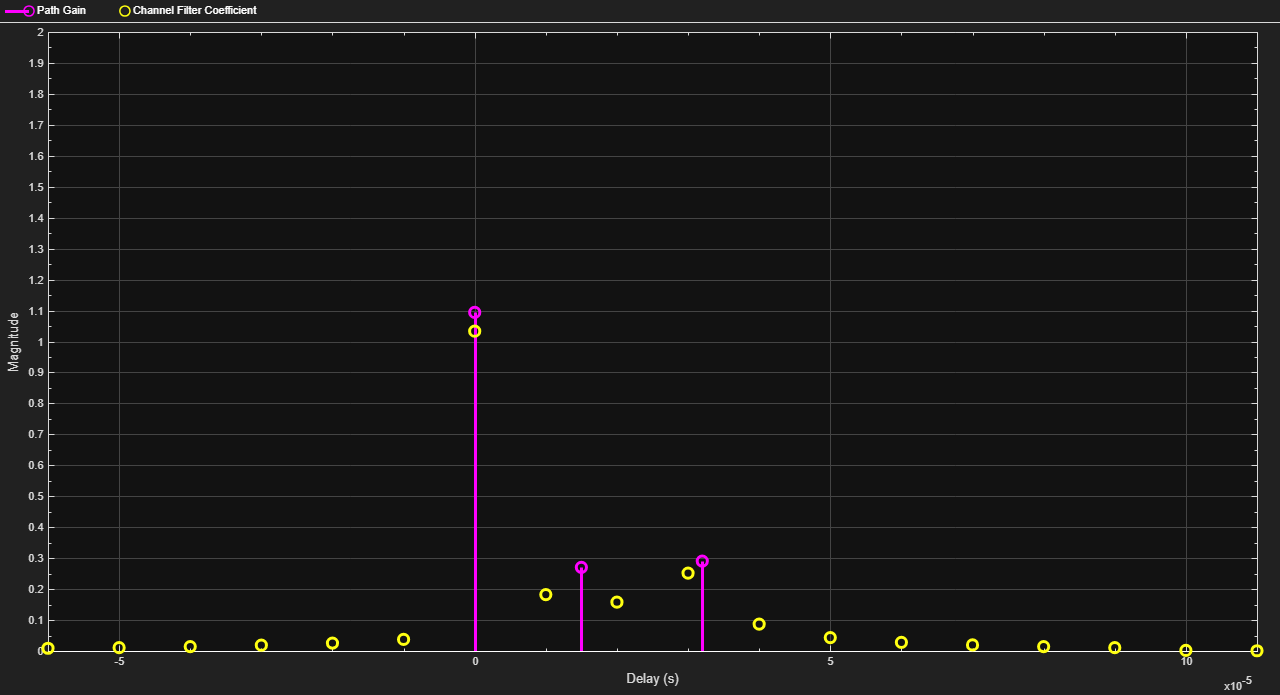The impulse response is plotted when the object is called.

This example plots the power of a faded signal versus sample number. It illustrates the syntax of creating and calling a `comm.RayleighChannel` fading channel object and the state retention of the channel object.

```rayChan = comm.RayleighChannel('SampleRate',10000,'MaximumDopplerShift',100); sig = j*ones(2000,1); % Signal out = rayChan(sig); % Pass signal through channel. rayChan % Display all properties of the channel object.```
```rayChan = comm.RayleighChannel with properties: SampleRate: 10000 PathDelays: 0 AveragePathGains: 0 NormalizePathGains: true MaximumDopplerShift: 100 DopplerSpectrum: [1x1 struct] Show all properties ```

Plot power of faded signal, versus sample number.

`plot(20*log10(abs(out)))`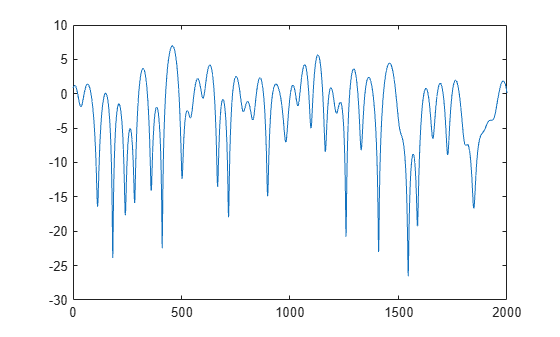#### DBPSK Empirical Versus Theoretical Performance in Fading Conditions

This example creates a frequency-flat Rayleigh fading channel object and calls it to process a DBPSK signal consisting of a single vector. Bit error rate is computed for different values of the signal-to-noise ratio. The fading channel filter is applied before AWGN. This is the recommended sequence to use when you combine fading with AWGN.

Create Rayleigh fading channel, modulator, and demodulator objects

`chan = comm.RayleighChannel('SampleRate',1e4,'MaximumDopplerShift',100);`

Create DBPSK modulator and demodulator objects with the modulation order set to `2`. Generate DBPSK modulated data and pass it through the channel.

```M = 2; % DBPSK modulation order tx = randi([0 M-1],50000,1); % Generate a random bit stream mod = comm.DBPSKModulator; demod = comm.DBPSKDemodulator; dpskSig = mod(tx); fadedSig = chan(dpskSig); % Apply the channel effects```

Create an AWGN channel object and an error rate calculator object.

```awgnChan = comm.AWGNChannel('NoiseMethod', 'Signal to noise ratio (SNR)'); errorCalc = comm.ErrorRate;```

Compute error rate for different values of SNR.

```SNR = 0:2:20; % Range of SNR values, in dB. numSNR = length(SNR); berVec = zeros(3, numSNR); % Preallocate a vector for BER results for n = 1:numSNR awgnChan.SNR = SNR(n); rxSig = awgnChan(fadedSig); % Add Gaussian noise rx = demod(rxSig); % Demodulate reset(errorCalc) berVec(:,n) = errorCalc(tx,rx); % Compute error rate. end BER = berVec(1,:);```

Compute theoretical performance results, for comparison.

`BERtheory = berfading(SNR,'dpsk',M,1);`

Plot BER results.

```semilogy(SNR,BERtheory,'b-',SNR,BER,'r*'); legend('Theoretical BER','Empirical BER'); xlabel('SNR (dB)'); ylabel('BER'); title('Binary DPSK over Rayleigh Fading Channel');```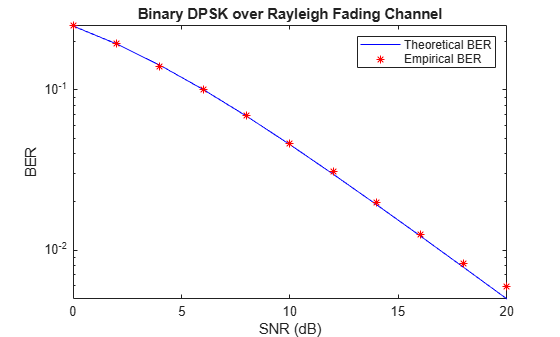#### Work with Channel Filter Delays

The value of a channel object's ChannelFilterDelay property is the number of samples by which the output of the channel lags the input. If you compare the input and output data sets directly, you must take the delay into account by using appropriate truncating or padding operations.

The example illustrates a way to account for the delay before computing a bit error rate.

Create DBPSK modulator and demodulator objects with the modulation order set to `2`. Generate DBPSK modulated data and pass it through the channel.

```bitRate = 50000; M = 2; % DQPSK modulation order mod = comm.DBPSKModulator; demod = comm.DBPSKDemodulator; ```

```rayChan = comm.RayleighChannel('SampleRate',bitRate,'MaximumDopplerShift',4,... 'PathDelays',[0 0.5/bitRate],'AveragePathGains',[0 -10]); chInfo = info(rayChan); delay = chInfo.ChannelFilterDelay;```

Generate random bit stream data. Modulate the data, pass it through the fading channel, and demodulate it.

```tx = randi([0 M-1],50000,1); dpskSig = mod(tx); fadedSig = rayChan(dpskSig); rx = demod(fadedSig); ```

Compute bit error rate, taking delay into account.

```errorCalc = comm.ErrorRate('ReceiveDelay', delay); berVec = step(errorCalc,tx,rx); ber = berVec(1)```
```ber = 0.0147 ```
`num = berVec(2)`
```num = 737 ```

#### Channel Filtering Using For Loop

This example filters input data through a Rayleigh fading channel within a `for` loop. It uses the small data sets from successive iterations to create an animated effect. The Rayleigh fading channel has two discrete major paths. For information on filtering data through a channel multiple times while maintaining continuity from one invocation to the next, see Configure Channel Objects Based on Simulation Needs.

Set up parameters. Specify a bit rate of `50e3` Hz, and a loop iteration count of `125`. Create a QPSK modulator and Rayleigh fading channel objects.

```bitRate = 50000; % Data rate is 50 kb/s numTrials = 125; % Number of iterations of loop M = 4; % QPSK modulation order qpskMod = comm.QPSKModulator; rayChan = comm.RayleighChannel('SampleRate',bitRate,'MaximumDopplerShift',4,'PathDelays',[0 2e-5],'AveragePathGains',[0 -9]); ```

Initialize a scatter plot.

```scatterPlot = comm.ConstellationDiagram; ```

Apply channel in a loop, maintaining continuity. Plot only the current data in each iteration.

```for n = 1:numTrials tx = randi([0 M-1],500,1); % Generate random bit stream pskSig = qpskMod(tx); % PSK modulate signal fadedSig = rayChan(pskSig); % Apply channel effects % Plot the new data from this iteration. update(scatterPlot,fadedSig); end ```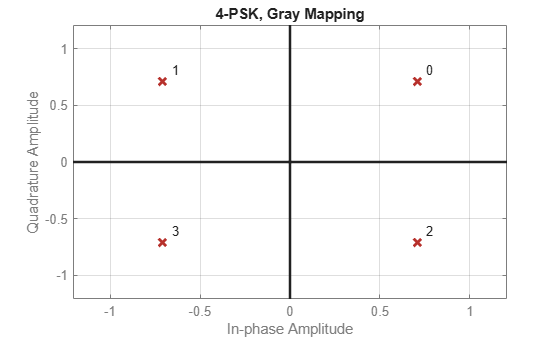#### Quasi-Static Channel Modeling

Typically, a path gain in a fading channel changes insignificantly over a period of `1/(100fd)` seconds, where `fd` is the maximum Doppler shift. Because this period corresponds to a very large number of bits in many modern wireless data applications, assessing performance over a statistically significant range of channel fading requires simulating a prohibitively large amount of data. This example illustrates the quasi-static channel modeling approach to gathering a statistically significant number of errors. Quasi-static channel modeling provides a more tractable approach, which you can implement using these steps:

1. Generate a random channel realization using a maximum Doppler shift of 0.

2. Process some large number of bits.

3. Compute error statistics.

4. Repeat these steps many times to produce a distribution of the performance metric.

Create modulator and demodulator system objects with a modulation order of `4`.

```M = 4; dpskMod = comm.DPSKModulator('ModulationOrder',M); dpskDemod = comm.DPSKDemodulator('ModulationOrder',M); numBits = 10000; % Each trial uses 10000 bits numTrials = 20; % Number of BER computations```

Typically, `numTrials` would be a large number to get an accurate estimate of outage probabilities or packet error rate. Use `20` here just to make the example run more quickly.

Create a Rician channel object and set the maximum Doppler shift to zero.

`ricianChan = comm.RicianChannel('KFactor',3,'MaximumDopplerShift',0);`

Within a `for` loop, generate a random bit stream, DPSK modulate the signal, filter the modulated signal through a Rician fading and AWGN channels, and demodulate the faded signal. For the symbol error rate computation on each packet, ignore the first sample because of DPSK initial condition.

```nErrors = zeros(1,numTrials); for n = 1:numTrials tx = randi([0 M-1],numBits,1); % Generate random bit stream dpskSig = dpskMod(tx); % DPSK modulate signal fadedSig = ricianChan(dpskSig); % Apply channel effects rxSig = awgn(fadedSig,15,'measured'); % Add Gaussian noise rx = dpskDemod(rxSig); % Demodulate nErrors(n) = symerr(tx(2:end),rx(2:end)); % Symbol error computation, beginning with sample index 2 end```

After the `for` loop ends, display the list of symbol error counts in the vector `nErrors` and a computation of the packet error rate. Run to run results vary due to randomness in the example.

`nErrors`
```nErrors = 1×20 0 0 1 0 0 0 0 0 0 0 0 0 0 0 0 0 0 0 0 0 ```
`per = mean(nErrors > 0) % Proportion of packets that had errors`
```per = 0.0500 ```

#### More About the Quasi-Static Technique

As an example to show how the quasi-static channel modeling approach can save computation, consider a wireless local area network (LAN) in which the carrier frequency is 2.4 GHz, mobile speed is 1 m/s, and bit rate is 10 Mb/s. The following expression shows that the channel changes insignificantly over 12,500 bits:

A traditional Monte Carlo approach for computing the error rate of this system would entail simulating thousands of times, totalling tens of millions of bits. By contrast, a quasi-static channel modeling approach would simulate a few packets at each of about 100 locations to arrive at a spatial distribution of error rates. From this distribution one could determine, for example, how reliable the communication link is for a random location within the indoor space. If each simulation contains 5,000 bits, 100 simulations would process half a million bits in total. This is substantially fewer bits compared to the traditional Monte Carlo approach.